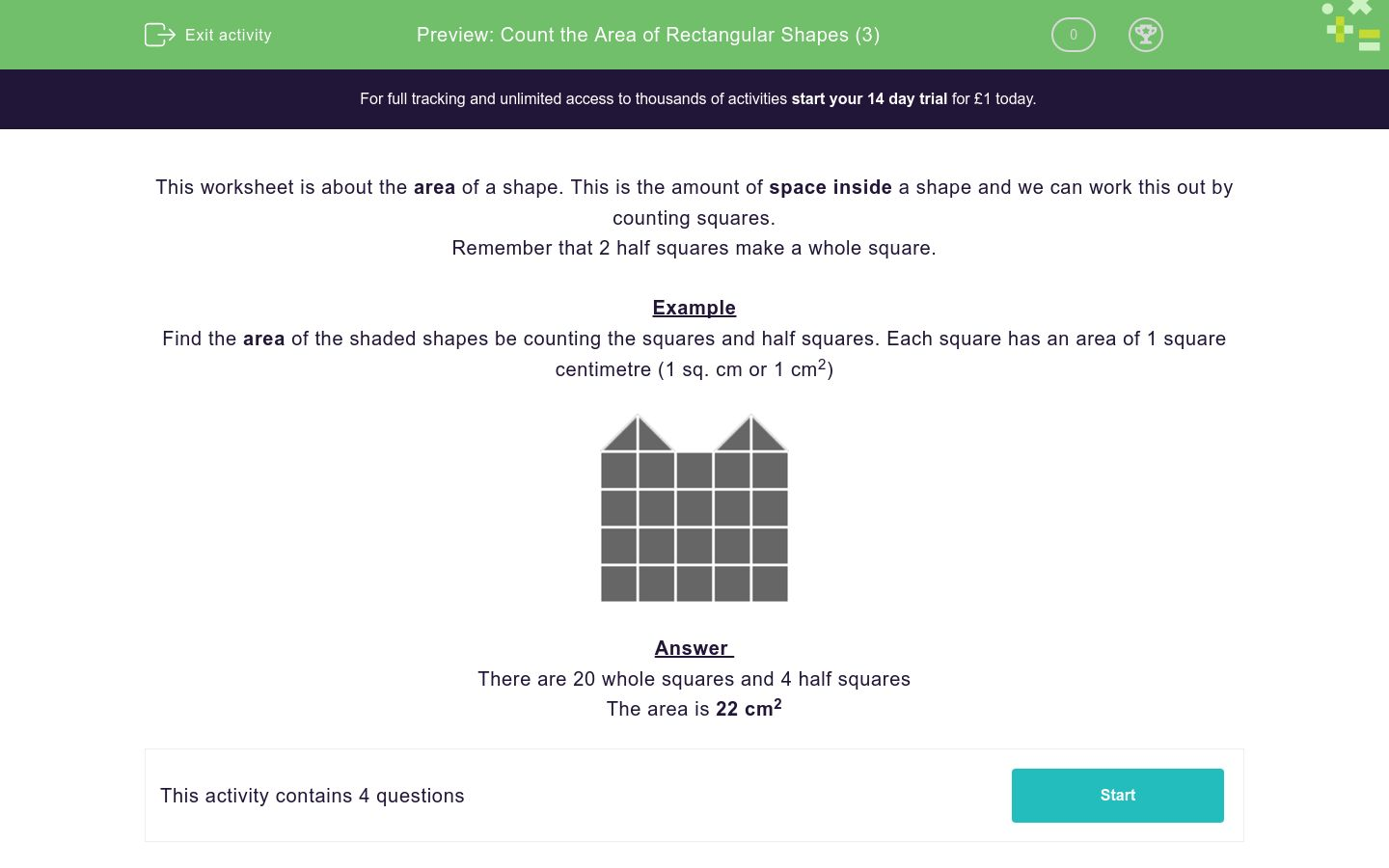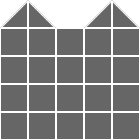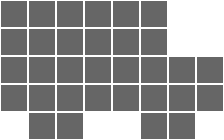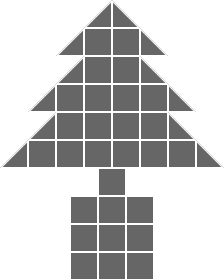Count the Area of Rectangular Shapes (3)

In this worksheet, students count squares and half squares to find the area of each 2D shape.Key stage:  KS 2

Curriculum topic:  Measurement

Curriculum subtopic:  Calculate Areas

Difficulty level:QUESTION 1 of 10

This worksheet is about the area of a shape. This is the amount of space inside a shape and we can work this out by counting squares.

Remember that 2 half squares make a whole square.

Example

Find the area of the shaded shapes be counting the squares and half squares. Each square has an area of 1 square centimetre (1 sq. cm or 1 cm2)There are 20 whole squares and 4 half squares

The area is 22 cm2

Find the area of the shaded shape by counting the squares and half squares.

Select the correct area in square centimetres below.30 cm2

32 cm

32 cm2

Find the area of the shaded shape by counting the squares and half squares.

Select the correct area in square centimetres below.33 cm2

31 cm2

32 cm2

Find the area of the shaded shape by counting the squares and half squares.

Select the correct area in square centimetres below.50 cm2

51 cm2

52 cm2

Find the area of the shaded shape by counting the squares and half squares.

Select the correct area in square centimetres below.34 cm2

35 cm2

32 cm2

• Question 1

Find the area of the shaded shape by counting the squares and half squares.

Select the correct area in square centimetres below.32 cm2
• Question 2

Find the area of the shaded shape by counting the squares and half squares.

Select the correct area in square centimetres below.31 cm2
• Question 3

Find the area of the shaded shape by counting the squares and half squares.

Select the correct area in square centimetres below.51 cm2
• Question 4

Find the area of the shaded shape by counting the squares and half squares.

Select the correct area in square centimetres below.